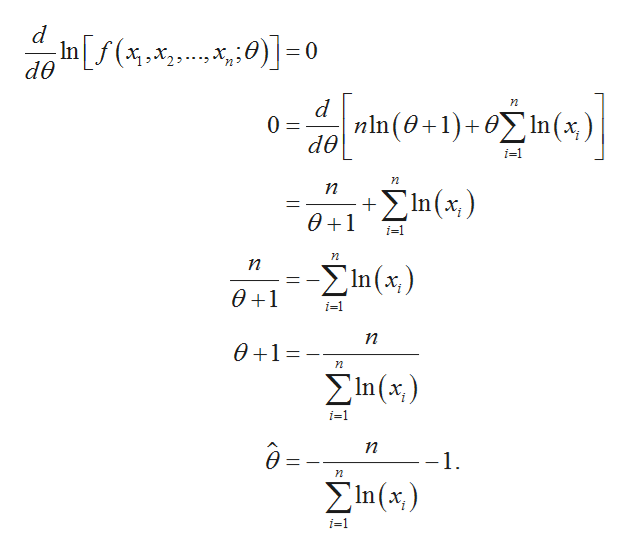# 1. Define a random variable X as the proportion of time that a student spends on a certain test. Research suggests that the pdf of x is f(x) = (θ + 1)xθ , 0 ≤ x ≤ 1. For the parameter θ, we know θ > −1, but its exact value is still unknown. To estimate the parameter, a random sample of 10 students is obtained, and their proportions of time are: 0.92, 0.79, 0.90, 0.65, 0.86, 0.47, 0.73, 0.97, 0.94, 0.77. Find the Maximum Likelihood Estimator (MLE) of θ.

Question
39 views

1. Define a random variable X as the proportion of time that a student spends on a certain test. Research suggests that the pdf of x is f(x) = (θ + 1)xθ , 0 ≤ x ≤ 1. For the parameter θ, we know θ > −1, but its exact value is still unknown. To estimate the parameter, a random sample of 10 students is obtained, and their proportions of time are: 0.92, 0.79, 0.90, 0.65, 0.86, 0.47, 0.73, 0.97, 0.94, 0.77. Find the Maximum Likelihood Estimator (MLE) of θ.

check_circle

Step 1

Likelihood function:

The pdf is considered as f(x)=(θ+1)xθ.

The likelihood function of the distribution is:

Step 2

Log likelihood function:

Thus, the log likelihood function obtained by taking logarithm of the likelihood function is:

Step 3

Differentiating the log likelihood function with respect to θ:

The maximum likelihood estimator of θ is obtained by differentiating the log ...help_outlineImage Transcriptionclosed In[f(x 0 2 de d 0 dθ η | nin (θ+1)+ ΟΣ 1n (x )| i=1 γη п ΣΠ(x)| θ+1 i-1 Pι п Σn (x) θ41 i-1 γη θ+1- PΟ Σn(x) i-1 δ. η -1 γη Σn(x) i-1 fullscreen

### Want to see the full answer?

See Solution

#### Want to see this answer and more?

Solutions are written by subject experts who are available 24/7. Questions are typically answered within 1 hour.*

See Solution
*Response times may vary by subject and question.
Tagged in

### Other